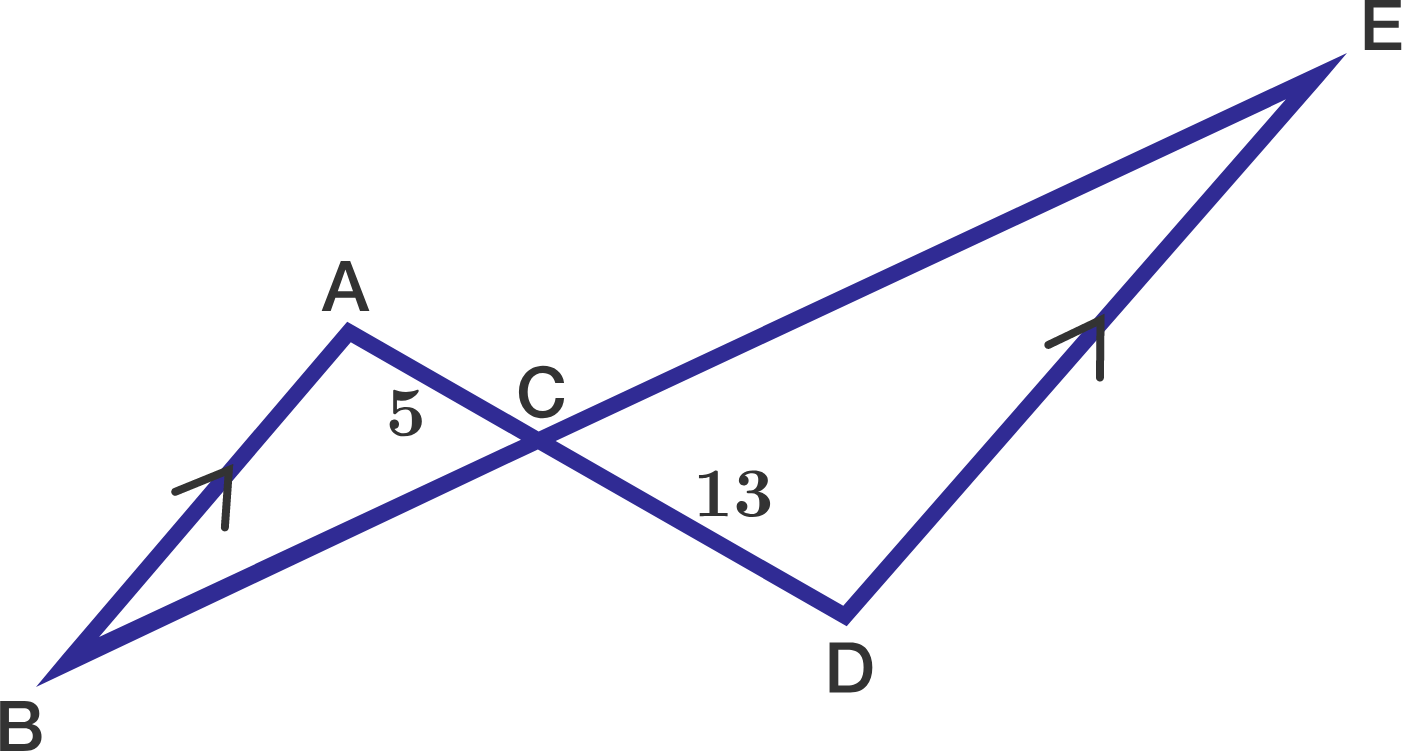# Similar Triangle Challenge

Geometry Level 1$\overline{AB}$ is parallel to $\overline{DE}$, $AC=5,$ and $CD=13.$

If the area of $\triangle ABC$ is $11,$ what is the area of $\triangle CDE$ ?

×

Problem Loading...

Note Loading...

Set Loading...